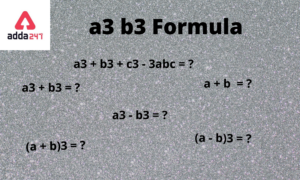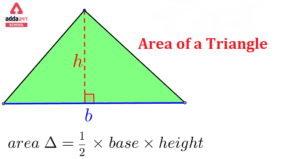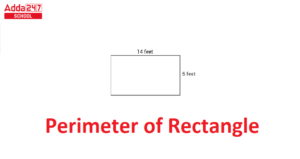Online Tution   »   Important Question   »   Slope Formula

# Slope Formula: Example in Maths & Coordinate Geometry

## Slope Formula

To calculate the steepness or inclination of the line we use the slope formula. The slope line shows how inclined the line is and to calculate the slope of lines we use the x and y coordinate of the line. The change in the y-axis ratio change in the x-axis is equal to the slope of the line.

## Slope Formula in Maths

The students find maths really difficult to understand they just ignore maths problems. To ease students who are facing issues in the topic Slope Formula, we are come up with this article to make students understand slope formula in laymen’s language. In this article, we have covered the slope formula and solved examples of slope formulas. Students must bookmark this page to get updates about related articles. We are updating here many related topics on daily basis.

## Slope Formula of Line in (Graphs) Maths & Coordinate Geometry

The slope formula is used to calculate the steepness of the line, in this formula the slope is computed as the change in y-axis ratio change in the x-axis. The computed slope will be zero if the line neither rises nor falls. The slope of the line will be defined as the line’s angle of inclination from the horizontal whether the slope rises or falls. The zero slopes occur when the horizontal lines neither rise nor fall which means the line extends till infinity in the left or right of the axis. The points in the coordinate geometry represent as a coordinate value. These points are found on the x-axis and y-axis, i.e. (x,y) represents the points on the geometric plane. Whereas the (0, 0) points represented the origin point of the coordinate.

## Slope Formula: Calculator Formula

Let’s derive the formula of the slope with the definition given above.

 m = (y2-y1)/(x2-x1)

Where,

x1 and x2 are the coordinates of the x-axis.

y1 and y2 are the coordinates of the y-axis.

m = slope of the line.

In the above formula, the numerator contains the difference of the points that exist on the y-axis, the points are y1 and y2. whereas the denominator contains the difference of the points that exist on the x-axis, the points are x1 and x2.

## Slope formula of a line

We only need two points from a line to find its slope: (x1, y1) and (x2, y2) (x2, y2). The slope of a straight line can be calculated in three steps.

Step 1: Find the two points on the line.

Step 2:  Identify one point to be (x1,y1)

Step 3: Identify another point to be (x2,y2).

Step 4: Use the slope formula given above to calculate the slope.

## Slope Formula: Example in Maths

Here we have given the solved examples of slope formula the students must try to solve the given questions without seeing their solutions, if unable to find the answer then refer to the given solution. Only mugging up concepts won’t work in the final examination, since the board rarely asks questions from theory in maths, so it won’t help much. Thus, students are advised to practice as much as questions they can. Try to solve the questions given below:

Example 1: Find the slope of the line whose coordinates are (4,2) and (7,9)?

Solution:

We have,

(x1, y1) = (4,2)

(x2, y2) = (7,9)

slope of a line formula  m = (y2 − y1) /( x2 − x1)

Put the values of x1, x2, y1, y2 in the above formula.

m = (9-2)/ (7-4)

m = 7/3

Here we have got the slope of line is 7/3.

Example 2: If the slope of a line passing through the points (2, a) and (4,  5) is 8, then what is the value of a?

Solution:

We have

Slope = m = 8

Points:

(x1, y1 ) = (2, a)

(x2, y2) = (4,  5)

We know that,

Slope (m) = (y2– y1 )/(x2– x1)

pull all the values in the slope formula given above

8 = 5-a/4-2

16 = 5-a

a = 5 – 16

a = -9

Therefore, the value of a = -9

Example 3: Find the slope of the line whose coordinates are (8, 6) and (9, 5)?

Solution:

We have,

(x1, y1) =(8, 6)

(x2, y2) = (9, 5)

slope of a line formula  m = (y2 − y1) /( x2 − x1)

Put the values of x1, x2, y1, y2 in the above formula.

m = (5-6)/ (9-8)

m = -1/1

m = -1

Here we have got the slope of line is -1.

Example 4: If the slope of a line passing through the points (6, 2) and (7,  x) is 4, then what is the value of x?

Solution:

We have

Slope = m = 4

Points:

(x1, y1 ) = (6, 2)

(x2, y2) = (7,  x)

We know that,

Slope (m) = (y2– y1 )/(x2– x1)

pull all the values in the slope formula given above

4 = x-2/7-6

4 = x-2/1

4 = x-2

x = 6

Therefore, the value of x = 6

Example 5: Find the slope of the line whose coordinates are (7, 6) and (1, 4)?

Solution:

We have,

(x1, y1) = (7, 6)

(x2, y2) = (1, 4)

slope of a line formula  m = (y2 − y1) /( x2 − x1)

Put the values of x1, x2, y1, y2 in the above formula.

m = (4-6)/ (1-7)

m = -2/(-6)

m = 1/3

Here we have got the slope of line is 1/3.

Example 6: If the slope of a line passing through the points (9, y) and (5,  2) is 4, then what is the value of y?

Solution:

We have

Slope = m = 4

Points:

(x1, y1 ) = (9, y)

(x2, y2) = (5,  2)

We know that,

Slope (m) = (y2– y1 )/(x2– x1)

pull all the values in the slope formula given above

4 = 2-y/5-9

4 = 2-y/(-4)

-16 = 2-y

y = 2+16

y = 18

Therefore, the value of y = 18

Related Posts:

## Slope Formula: FAQs

Q. What is the slope formula in maths?

The change in the y-axis ratio change in the x-axis is equal to the slope of the line.

Slope (m) = (y2– y1 )/(x2– x1)

Q. What is slope of line?

The slope line shows how inclined the line is and to calculate the slope of lines we use the x and y coordinate of the line. The change in the y-axis ratio change in the x-axis is equal to the slope of the line.

Q. What does m stand for in the slope formula?

The m stands for the slope of the line in the slope formula.

Q. What is the use of the slope formula?

The slope formula is used to calculate the steepness or inclination of the line.

Sharing is caring!

## FAQs

### Q. What is the slope formula in maths?

The change in the y-axis ratio change in the x-axis is equal to the slope of the line.
Slope (m) = (y2– y1 )/(x2– x1)

### Q. What is slope of line?

The slope line shows how inclined the line is and to calculate the slope of lines we use the x and y coordinate of the line. The change in the y-axis ratio change in the x-axis is equal to the slope of the line.

### Q. What does m stand for in the slope formula?

The m stands for the slope of the line in the slope formula.

### Q. What is the use of the slope formula?

The slope formula is used to calculate the steepness or inclination of the line.

Thank You, Your details have been submitted we will get back to you.

### TOPICS:

•Decomposition Reaction, Examples, Formul...
•a3 b3 Formula- Proof, Solution and Examp...
•Area of Triangle- Formula in Class 10 Co...
•Perimeter of Rectangle Formula Unit, Que...
•Compound Interest Formula, Definition, C...
•Force Formula Physics- Definition, Examp...